# ICSE Class 10 Mathematics 2018 Sample Paper

Class 10 Maths

You have to attempt all questions from Section A and any four questions from Section B.

### SECTION A (40 Marks)

Question 1

(a) Find the value of ‘k’ if 4𝑥3 − 2𝑥2 + 𝑘𝑥 + 5 leaves remainder -10 when divided by 2𝑥 + 1. 

(b) Amit deposits Rs. 1600 per month in a bank for 18 months in a recurring deposit account. If he gets Rs. 31,080 at the time of maturity, what is the rate of interest per annum? 

(c) The price of an article is Rs. 9350 which includes VAT at 10%. Find how much less a customer pays for the article, if the VAT on the article decreases by 3%. 

Question 2

(a) Solve the following inequation and represent your solution on the real number line: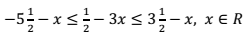(b) Find the 16th term of the A.P. 7, 11, 15, 19…. Find the sum of the first 6 terms. 

(c) In the given figure CE is a tangent to the circle at point C. ABCD is a cyclic quadrilateral. If ∠ABC = 93° and ∠DCE = 35°.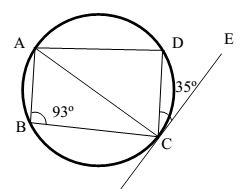Find:
(ii) ∠ACD

Question 3

(a) Prove the following identity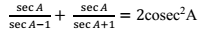(b) Find x and y if: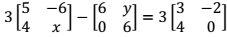(c) For what value of ‘k’ will the following quadratic equation:
(𝑘 + 1)𝑥2 − 4𝑘𝑥 + 9 = 0 have real and equal roots? Solve the equations. 

Question 4

(a) A box consists of 4 red, 5 black and 6 white balls. One ball is drawn out at random. Find the probability that the ball drawn is:
(i) black
(ii) red or white 

(b) Calculate the median and mode for the following distribution: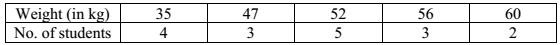(c) A solid cylinder of radius 7 cm and height 14 cm is melted and recast into solid spheres each of radius 3.5 cm. Find the number of spheres formed. 

### SECTION B (40 Marks)

Question 5

(a) The 2nd and 45th term of an arithmetic progression are 10 and 96 respectively. Find the first term and the common difference and hence find the sum of the first 15 terms. 

(b) If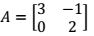, find matrix B such that A2 – 2B = 3A + 5I where I is a 2 x 2 identity matrix. 

(c) With the help of a graph paper, taking 1 cm = 1 unit along both x and y axis: 

(i) Plot points A (0, 3), B (2, 3), C (3, 0), D (2, -3), E (0, -3)

(ii) Reflect points B, C and D on the y axis and name them as B', C' and D' respectively.

(iii) Write the co-ordinates of B', C' and D'.

(iv) Write the equation of line B' D'.

(v) Name the figure BCDD'C'B'B

Question 6

(a) In ∆ABC and ∆EDC, AB is parallel to ED. BD = 1/3 BC and AB = 12.3 cm. 

(i) Prove that ∆ABC ~ ∆EDC.

(ii) Find DE

(iii) Find: 𝑎𝑟𝑒𝑎 𝑜𝑓 ∆𝐸𝐷𝐶/𝑎𝑟𝑒𝑎 𝑜𝑓 ∆𝐴𝐵𝐶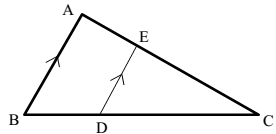(b) Find the ratio in which the line joining (-2, 5) and (-5, -6) is divided by the line y = -3. Hence find the point of intersection. 

(c) The given solid figure is a cylinder surmounted by a cone. The diameter of the base of the cylinder is 6 cm. The height of the cone is 4 cm and the total height of the solid is 25 cm. Take π = 22/7.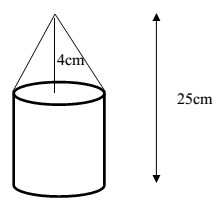Find the:

(i) Volume of the solid

(ii) Curved surface area of the solid

Question 7

(a) In the given figure, PAB is a secant and PT a tangent to the circle with centre O. If ∠ATP = 40°, PA = 9 cm and AB = 7 cm.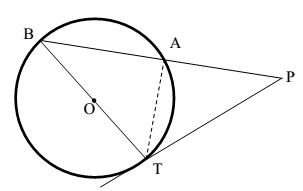Find:
(i) ∠APT
(ii) length of PT

(b) The 1st and the 8th term of a GP are 4 and 512 respectively. Find:
(i) the common ratio
(ii) the sum of its first 5 terms. 

(c) The mean of the following distribution is 49. Find the missing frequency ‘a’.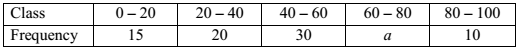Question 8

(a) Prove the following identity

(sinA + cosecA)2 + (cosA + secA)2 = 5 + sec2A . cosec2A 

(b) Find the equation of the perpendicular bisector of line segment joining A(4, 2) and B(-3, -5) 

(c) Using properties of proportion, find x : y if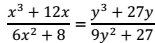Question 9

(a) The difference of the squares of two natural numbers is 84. The square of the larger number is 25 times the smaller number. Find the numbers. 

(b) The following table shows the distribution of marks in Mathematics: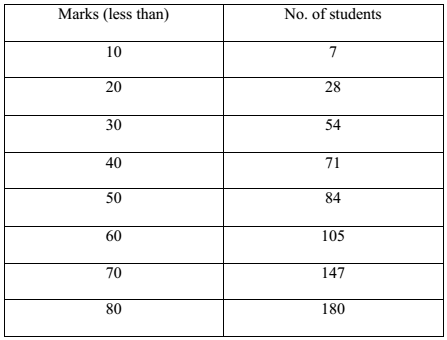With the help of a graph paper, taking 2 cm = 10 units along one axis and 2 cm = 20 units along the other axis, plot an ogive for the above distribution and use it to find the:

(i) median.

(ii) number of students who scored distinction marks (75% and above)

(iii) number of students, who passed the examination if pass marks is 35%.

Question 10

(a) Prove that two tangents drawn from an external point to a circle are of equal length. 

(b) From the given figure find the: 

(i) Coordinates of points P, Q, R.

(ii) Equation of the line through P and parallel to QR.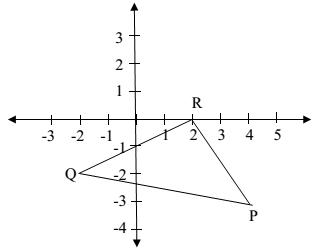(c) A manufacturer sells an article to a wholesaler with marked price Rs. 2000 at a discount of 20% on the marked price. The wholesaler sells it to a retailer at a discount of 12% on the marked price. The retailer sells the article at the marked price. If the VAT paid by the wholesaler is Rs. 11.20, find the: 

(i) Rate of VAT

(ii) VAT paid by the retailer.

Question 11

(a) Mr. Sharma receives an annual income of Rs. 900 in buying Rs. 50 shares selling at Rs. 80. If the dividend declared is 20%, find the: 

(i) Amount invested by Mr. Sharma.

(ii) Percentage return on his investment.

(b) Two poles AB and PQ are standing opposite each other on either side of a road 200 m wide. From a point R between them on the road, the angles of elevation of the top of the poles AB and PQ are 45° and 40° respectively. If height of AB = 80 m, find the height of PQ correct to the nearest metre. 

(c) Construct a triangle PQR, given RQ = 10 cm, ∠PRQ = 75° and base RP = 8 cm. 

Find by construction:

(i) The locus of points which are equidistant from QR and QP.

(ii) The locus of points which are equidistant from P and Q.

(iii) Mark the point O which satisfies conditions (i) and (ii).